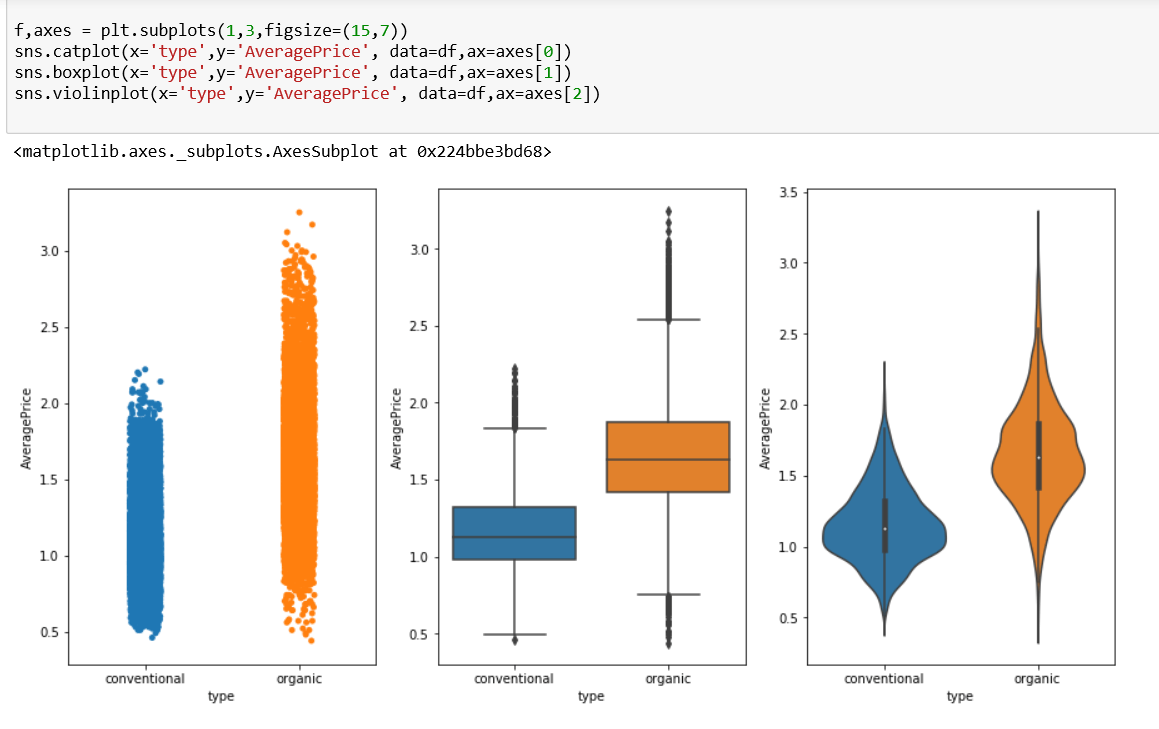# Seaborn Visuals Quick Guide

Seaborn is one the best statistical visual libraries that Python has to offer. Here is a quick guide that will allow you quick produce visuals easily. For this guide I am using a Avocado data set which you can find below. Check out how to quickly become a data viz master with Data Visualization at Datacamp.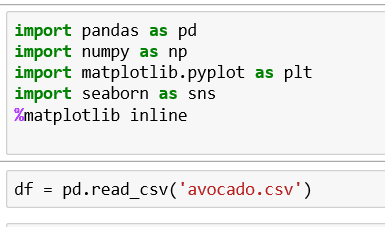If you are not familiar with Pandas. Check out our Pandas quick start guide.

### Bar Plots

Barplots can easily be created by defining the x and y variables and the actual data variable.  You can change the orientation of the barplot by add orient =’h’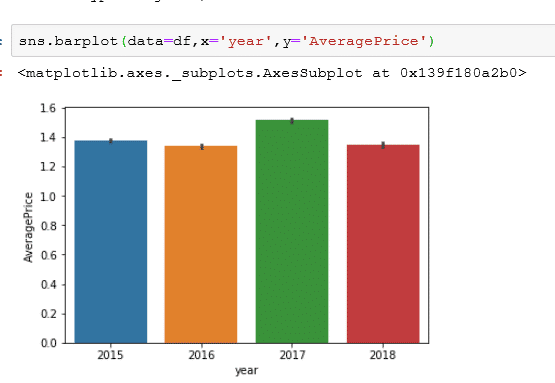### Line Plots

Line plots are essential and only require the x and y variables. However, you can use a time series plot by adding a datetime value to the x variable.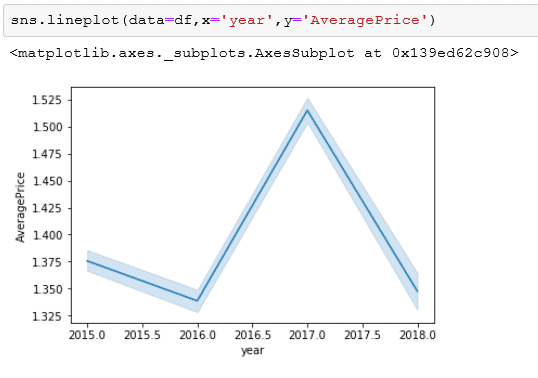For more on styling line charts with Seaborn

### Scatter Plots

You can evaluate two continuous variables by using a scatter plot. You can further customize this by using the hue parameter. For more details on styling scatter plots in Seaborn check out this Seaborn scatter plot tutorial.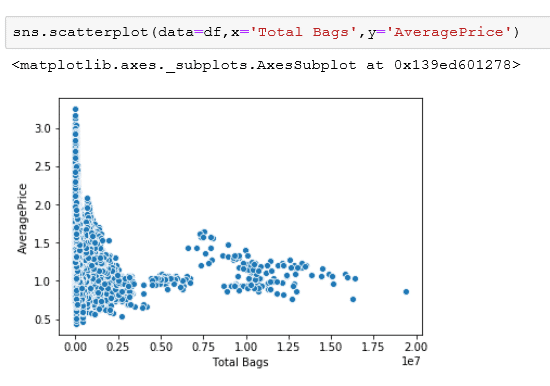### Regression Plots

A modified version of a scatterplot is a regression plot that allows you to have a clear line of best fit which is based on the regression function.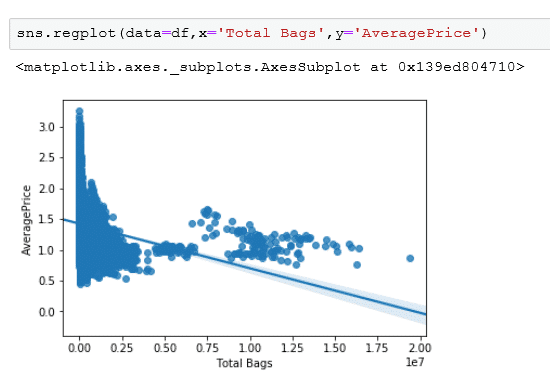### Box Plots

Box plots are great way to evaluate the centrality of your data and find outliers. This follows the typical parameters needed for Seaborn which are defining the X and Y variables and the data source.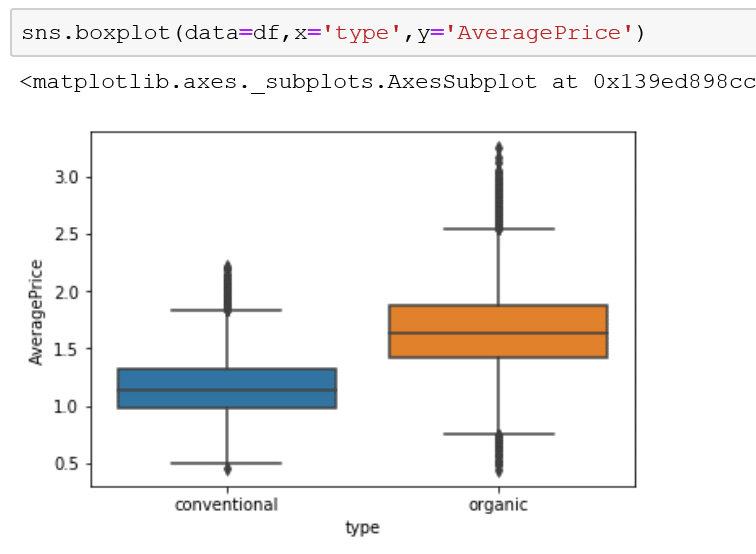### Category Plot

A category plot is a modified bar plot that can visualize the raw density of the data underlying data.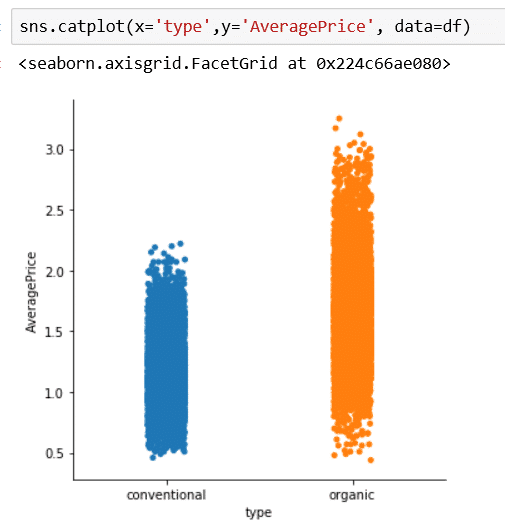### Violin Plot

A violin plot is the cousin to a box plot. However, the density of the data is distributed along the the y axis.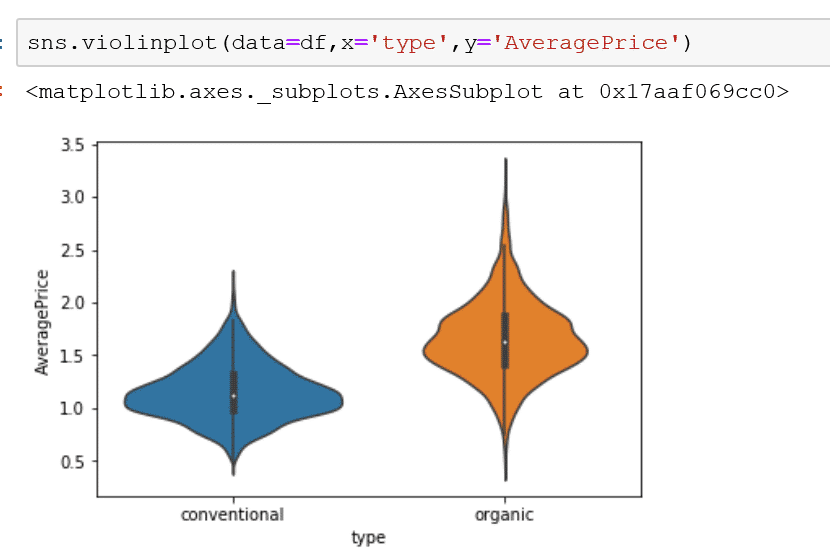### Histogram(Seaborn Displot)

This plot allows you to to see the frequency of your values distributed in bins. Therefore only a single value is needed to produce this plot.

How to style a Seaborn Histogram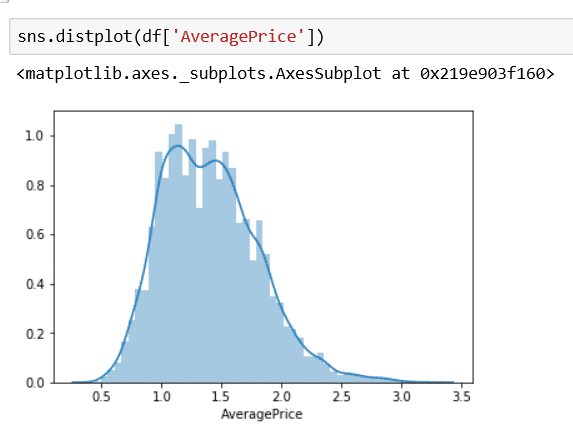### Joint Plot

Join plot allows you to produce a scatter plot and produce a density with marginal histograms.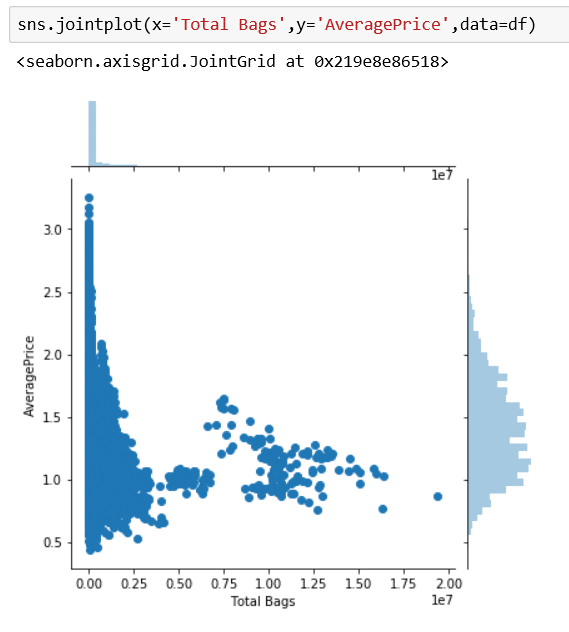### Heatmap

One of the best ways to see correlation is to use the seaborn heatmap. In order to achieve this you need to produce a correlation of the existing dataframe.  You will need to add the corr() function to a data frame.

How to Style a Seaborn Heatmap### Pair plot

A pairplot will show a collective correlation of variables in subplots. This function takes a single variable which is the data frame.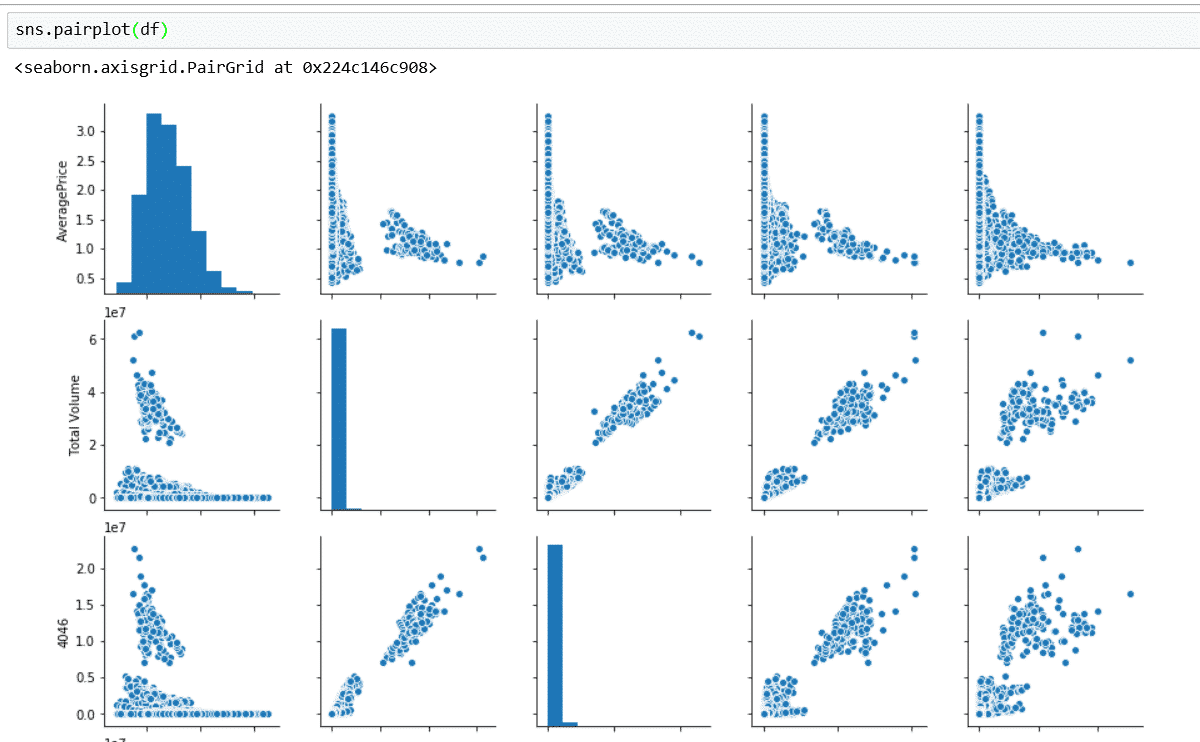### How Create Sublplots in Seaborn

To create a subplots, you are going to eventually chain together your plots by initiating a maplotlib function to set up your subplots. You will need to declare the structure for example the 3,0 parameter points out that there are 3 columns and no rows. you can change this to the structure that best fits your subplots.